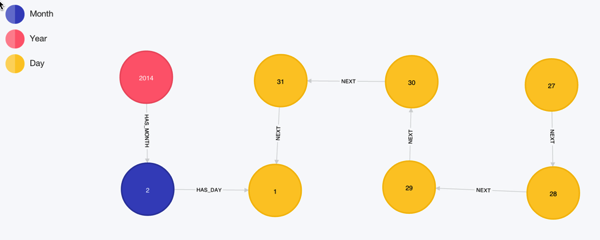# Neo4j: Cypher - Creating a time tree down to the day

Michael recently wrote a blog post showing how to create a time tree representing time down to the second using Neo4j's Cypher query language, something I built on top of for a side project I'm working on.

The domain I want to model is RSVPs to meetup invites - I want to understand how much in advance people respond and how likely they are to drop out at a later stage.

For this problem I only need to measure time down to the day so my task is a bit easier than Michael's.

After a bit of fiddling around with leap years I believe the following query will create a time tree representing all the days from 2011 - 2014, which covers the time the London Neo4j meetup has been running:

``````
WITH range(2011, 2014) AS years, range(1,12) as months
FOREACH(year IN years |
MERGE (y:Year {year: year})
FOREACH(month IN months |
CREATE (m:Month {month: month})
MERGE (y)-[:HAS_MONTH]->(m)
FOREACH(day IN (CASE
WHEN month IN [1,3,5,7,8,10,12] THEN range(1,31)
WHEN month = 2 THEN
CASE
WHEN year % 4 <> 0 THEN range(1,28)
WHEN year % 100 <> 0 THEN range(1,29)
WHEN year % 400 = 0 THEN range(1,29)
ELSE range(1,28)
END
ELSE range(1,30)
END) |
CREATE (d:Day {day: day})
MERGE (m)-[:HAS_DAY]->(d))))
``````

The next step is to link adjacent days together so that we can easily traverse between adjacent days without needing to go back up and down the tree. For example we should have something like this:

``````
(jan31)-[:NEXT]->(feb1)-[:NEXT]->(feb2)
``````

We can build this by first collecting all the 'day' nodes in date order like so:

``````
MATCH (year:Year)-[:HAS_MONTH]->(month)-[:HAS_DAY]->(day)
WITH year,month,day
ORDER BY year.year, month.month, day.day
WITH collect(day) as days
RETURN days
``````

And then iterating over adjacent nodes to create the 'NEXT' relationship:

``````
MATCH (year:Year)-[:HAS_MONTH]->(month)-[:HAS_DAY]->(day)
WITH year,month,day
ORDER BY year.year, month.month, day.day
WITH collect(day) as days
FOREACH(i in RANGE(0, length(days)-2) |
FOREACH(day1 in [days[i]] |
FOREACH(day2 in [days[i+1]] |
CREATE UNIQUE (day1)-[:NEXT]->(day2))))
``````

Now if we want to find the previous 5 days from the 1st February 2014 we could write the following query:

``````
MATCH (y:Year {year: 2014})-[:HAS_MONTH]->(m:Month {month: 2})-[:HAS_DAY]->(:Day {day: 1})<-[:NEXT*0..5]-(day)
RETURN y,m,day
``````If we want to we can create the time tree and then connect the day nodes all in one query by using 'WITH *' like so:

``````
WITH range(2011, 2014) AS years, range(1,12) as months
FOREACH(year IN years |
MERGE (y:Year {year: year})
FOREACH(month IN months |
CREATE (m:Month {month: month})
MERGE (y)-[:HAS_MONTH]->(m)
FOREACH(day IN (CASE
WHEN month IN [1,3,5,7,8,10,12] THEN range(1,31)
WHEN month = 2 THEN
CASE
WHEN year % 4 <> 0 THEN range(1,28)
WHEN year % 100 <> 0 THEN range(1,29)
WHEN year % 400 = 0 THEN range(1,29)
ELSE range(1,28)
END
ELSE range(1,30)
END) |
CREATE (d:Day {day: day})
MERGE (m)-[:HAS_DAY]->(d))))

WITH *

MATCH (year:Year)-[:HAS_MONTH]->(month)-[:HAS_DAY]->(day)
WITH year,month,day
ORDER BY year.year, month.month, day.day
WITH collect(day) as days
FOREACH(i in RANGE(0, length(days)-2) |
FOREACH(day1 in [days[i]] |
FOREACH(day2 in [days[i+1]] |
CREATE UNIQUE (day1)-[:NEXT]->(day2))))
``````

Now I need to connect the RSVP events to the tree!

### Updated: 13th August 2017

I updated the Cypher query to fix a bug pointed out in the comments by Adam Hill and Colin.